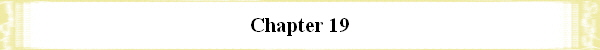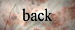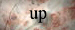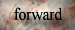THE  NAME  OF  GOD  AND  NUMBERS

The Hebrew characters or letters all have the meaning of numbers because they were also used as numbers. IOUO means 26, because I = 10, O = 5, U = 6. The number 26 recalls to mind the 13, because 26 = 2 times 13. 13 is often used in the Bible: Actually 13 Israelite tribes (Levi + 12 tribes), Jesus and 12 disciples = 13, Jacob and 12 sons. The double of 26 is biblical, too: 26 times 2 =  52. Nehemiah needed 52 days to construct Jerusalem's walls. 52 days were from Nisan 14, 33 C.E., the death of Jesus, till the pouring out of the holy spirit in Pentecost 33 C.E. (first day and last day included).

The name AOUO means 17 (A = 1, O = 5, U = 6) a prime number. The name TOUO means 416 (T = 400, O = 5, U = 6). 416 = 16 times 26 = 42 times 26 = 22 times 22 times 26, so TOUO is two times a square number times the  meaning of IOUO, a square being a holy number.

In Greek it is the same: ÍOUO means 550 (I = 10, O = 70, Y = 400). ÍWUW means 2010 (I = 10, W =  800, Y = 400). TOYO means 840 (T = 300, O = 70, Y = 400). 840 is divisible by 7 and by 12, two biblical numbers. AOYO means 541 (A = 1).

IOYO can also be counted after the place-numbers in the alphabet, let's try what we get then! I = 10, O = 16, Y = 21 (Number 6 is a missing place in the Greek alphabet; it is the Digamma), then we come to 63 for IOYO. 63 = 32 times 7, that is a square number times the holy number 7.

Dr. Ivan Panin investigated the Bible and its number-meanings of all words.(47) He especially investigated the division by the number 7. It would be interesting to make an investigation also of other numbers (like 3, 4, 5, 6, 8, 12, 13, 24, 40, 49, 50). Especially the numbers of the name of God are of interest (26, 17, 416, 550, 2010, 840, 541). Not only the division should be investigated but also other mathematical functions.

(47) Erstaunliche neue Entdeckungen, by Karl G. Sabiers, Exodus Verlag J. Henning, translated from English[Home] [Introduction] [Study] [Download] [Feedback](c) Kurt M. Niedenfuehr 2001 - All Rights Reserved